Code Chunk

Markdown Preview Enhanced 支持渲染代码的运行结果。

1. bash {cmd=true}
2. ls .
3. 
4. javascript {cmd="node"}
5. const date = Date.now()
6. console.log(date.toString())
7. 

命令 & 快捷键

• Markdown Preview Enhanced: Run Code Chunk 或者 shift-enter
运行你现在光标所在的一个 code chunk。
• Markdown Preview Enhanced: Run All Code Chunks 或者 ctrl-shift-enter
运行所有的 code chunks。

格式

lang {cmd=你的命令 opt1=value1 opt2=value2 …}

lang

基本设置

cmd

1. python {cmd="/usr/local/bin/python3"}
2. print("这个将会运行 python3 程序")
3. 

output
html, markdown, text, png, none

html 将会添加输出结果为 html。
markdown 将会添加输出结果为 markdown。（MathJax 以及 graphs 在这种情况下是不被支持的）
text 将会添加输出结果到 pre 块。
png 将会添加输出结果到 base64 图片。
none 将会隐藏输出结果。

1. gnuplot {cmd=true output="html"}
2. set terminal svg
3. set title "Simple Plots" font ",20"
4. set key left box
5. set samples 50
6. set style data points
7. plot [-10:10] sin(x),atan(x),cos(atan(x))
8.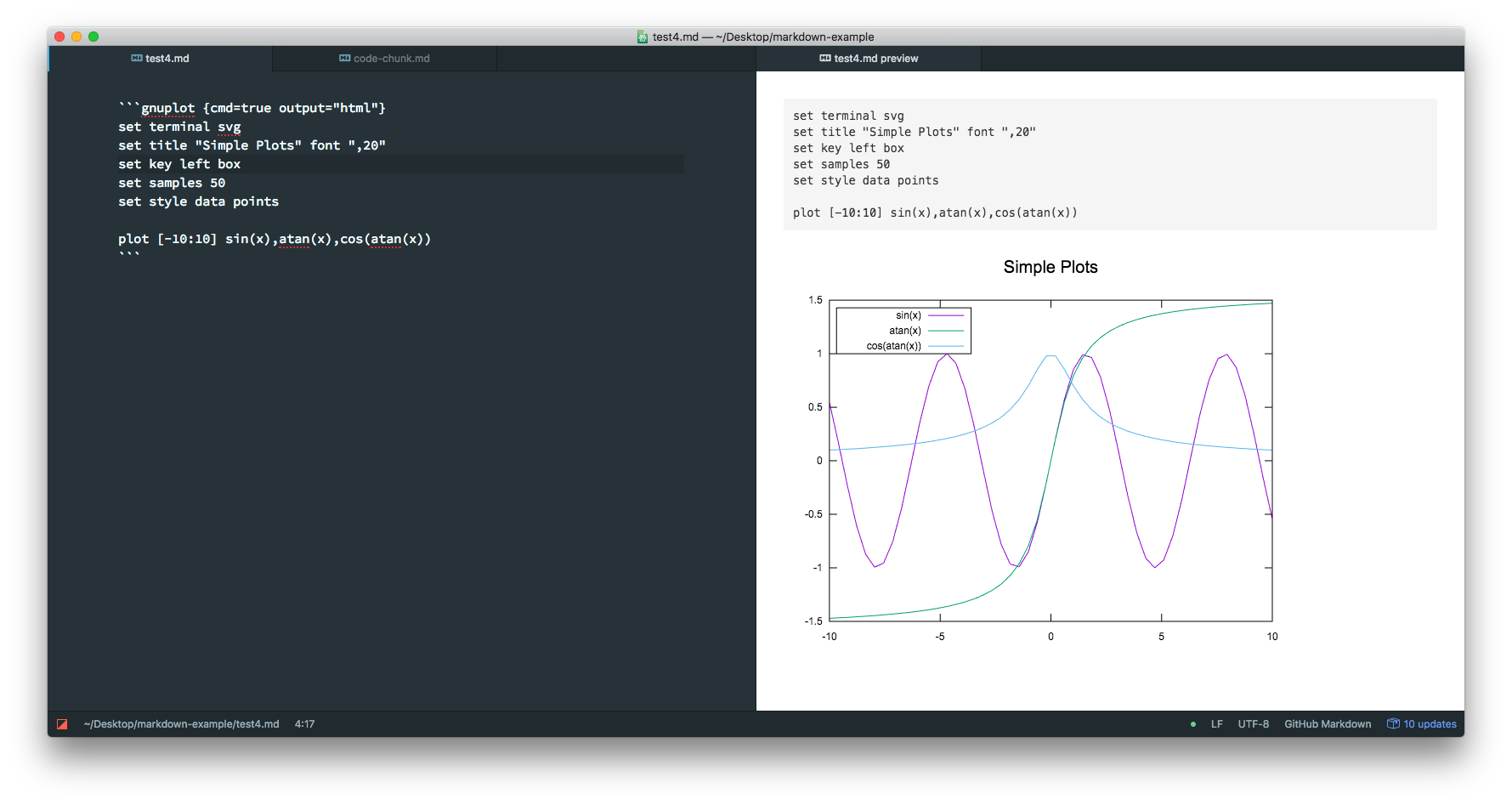args

1. python {cmd=true args=["-v"]}
2. print("Verbose will be printed first")
3. 
4. erd {cmd=true args=["-i", "$input_file", "-f", "svg"] output="html"} 5. # output svg format and append as html result. 6.  stdin 如果 stdin 被设置为 true，那么代码将会被传递到 stdin 而不是作为文件。 hide hide 将会隐藏代码块但是会显示运行结果，默认为 false 例如： 1. python {hide=true} 2. print('you can see this output message, but not this code') 3.  continue 如果设置 continue: true，那么这个 code chunk 将会继续运行上一个 code chunk 的内容。 如果设置continue: id，那么这个 code chunk 从拥有这个 id 的 code chunk 运行。 例如： 1. python {cmd=true id="izdlk700"} 2. x = 1 3.  4. python {cmd=true id="izdlkdim"} 5. x = 2 6.  7. python {cmd=true continue="izdlk700" id="izdlkhso"} 8. print(x) # will print 1 9.  class 如果设置 class="class1 class2"，那么 class1 class2 将会被添加到 code chunk。 • line-numbers class 将会添加代码行数到 code chunk。 element 你想要添加的元素。 请查看下面的 Plotly 例子。 run_on_save boolean 当 markdown 文件被保存时，自动运行 code chunk。默认 false modify_source boolean 插入 code chunk 的运行结果直接到 markdown 文件。默认 false id Code chunk 的 id。这个选项可以配合 continue 选项使用。 宏 • input_file input_file 将会拷贝你的 code chunk 中的代码，然后在你的 markdown 文件的目录下生成一个临时文件，并且会在 code chunk 运行结束后被自动删除。 默认条件下，它被作为程序运行的最后一个参数。 但是，如果你想要改变 input_file 在你的 args 中的位置，你可以使用$input_file 宏。例如：
1. program {cmd=true args=["-i", "$input_file", "-o", "./output.png"]} 2. ...your code here 3.  Matplotlib 如果设置 matplotlib=true，那么你的 python code chunk 将会在你的预览中绘制图像。 例如： 1. python {cmd=true matplotlib=true} 2. import matplotlib.pyplot as plt 3. plt.plot([1,2,3, 4]) 4. plt.show() # show figure 5.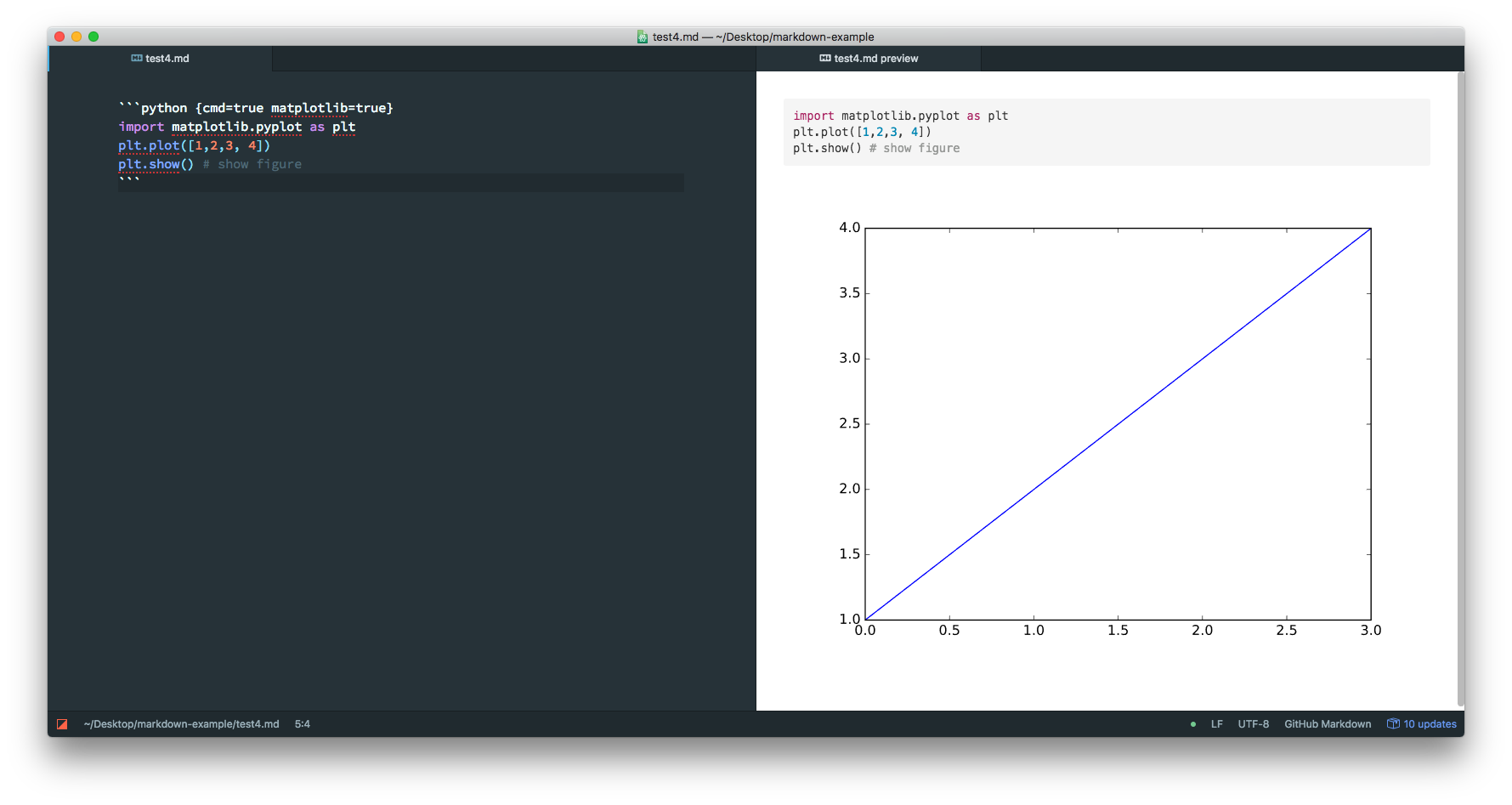LaTeX Markdown Preview Enhanced 也支持 LaTeX 编译。 在使用这个特性之前，你需要先安装好 pdf2svg 以及 LaTeX engine 然后你就可以很简单的利用 code chunk 编写 LaTeX 了： 1. latex {cmd=true} 2. \documentclass{standalone} 3. \begin{document} 4. Hello world! 5. \end{document} 6.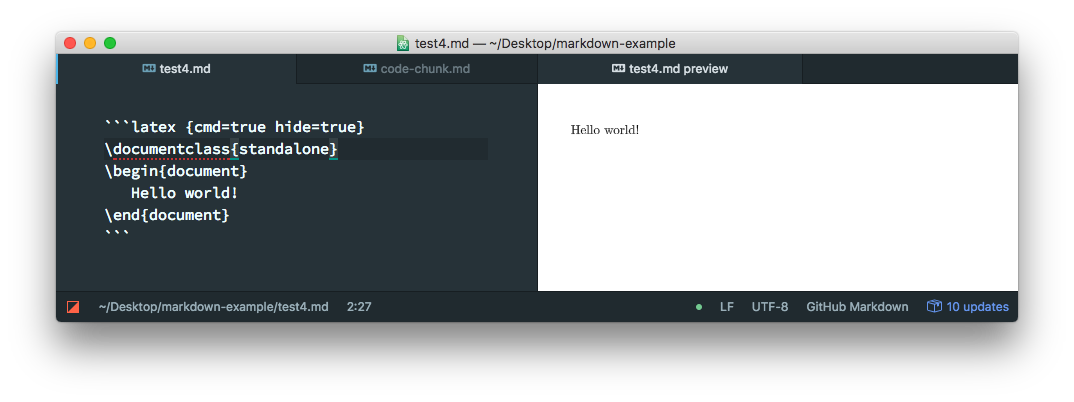LaTeX 输出设置 latex_zoom 如果设置了 latex_zoom=num，那么输出结果将会被缩放 num 倍。 latex_width 输出结果的宽度。 latex_height 输出结果的高度。 latex_engine 就会被用来编译 tex 文件的 latex 引擎。默认下 pdflatex 是被使用的。你可以在 插件设置 中改变它的默认值。 TikZ 例子 推荐使用 standalone 绘制 tikz 图形。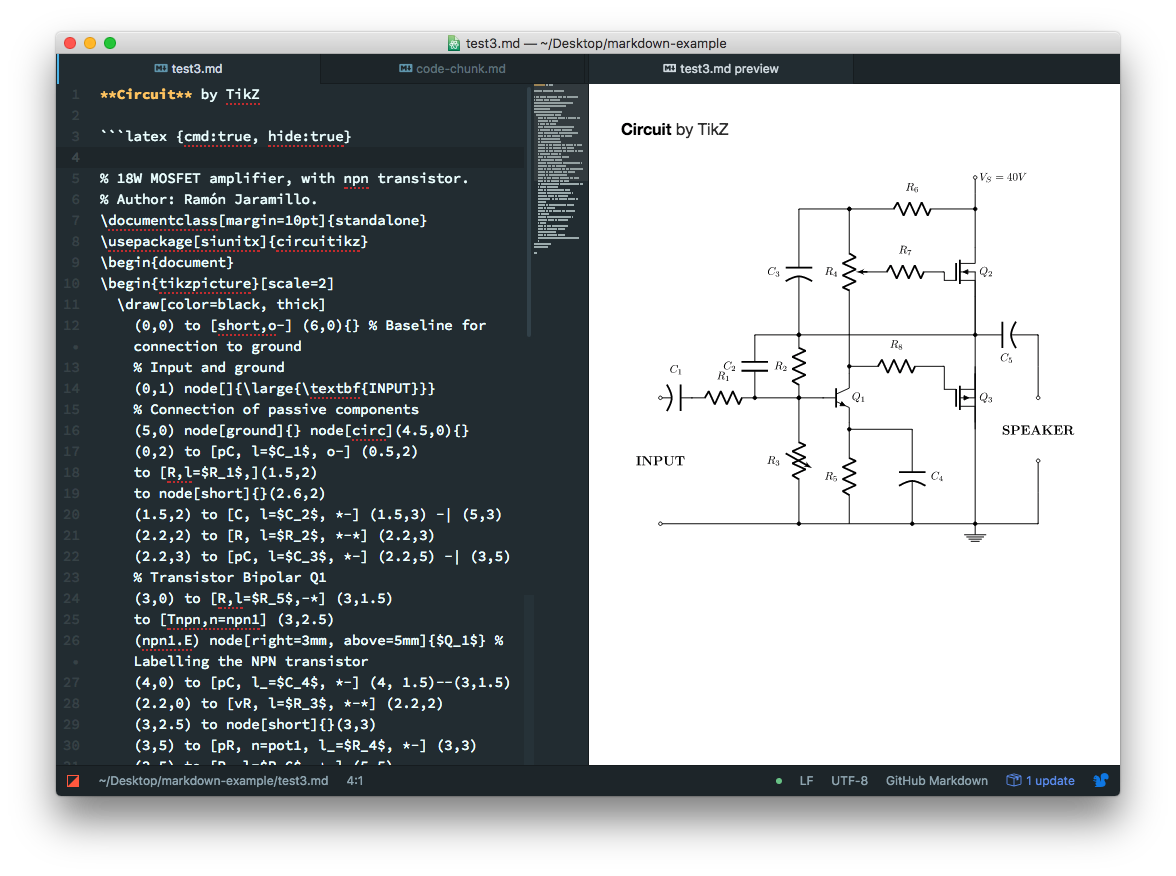Plotly Markdown Preview Enhanced 支持你轻松的绘制 Plotly 图形。 例如：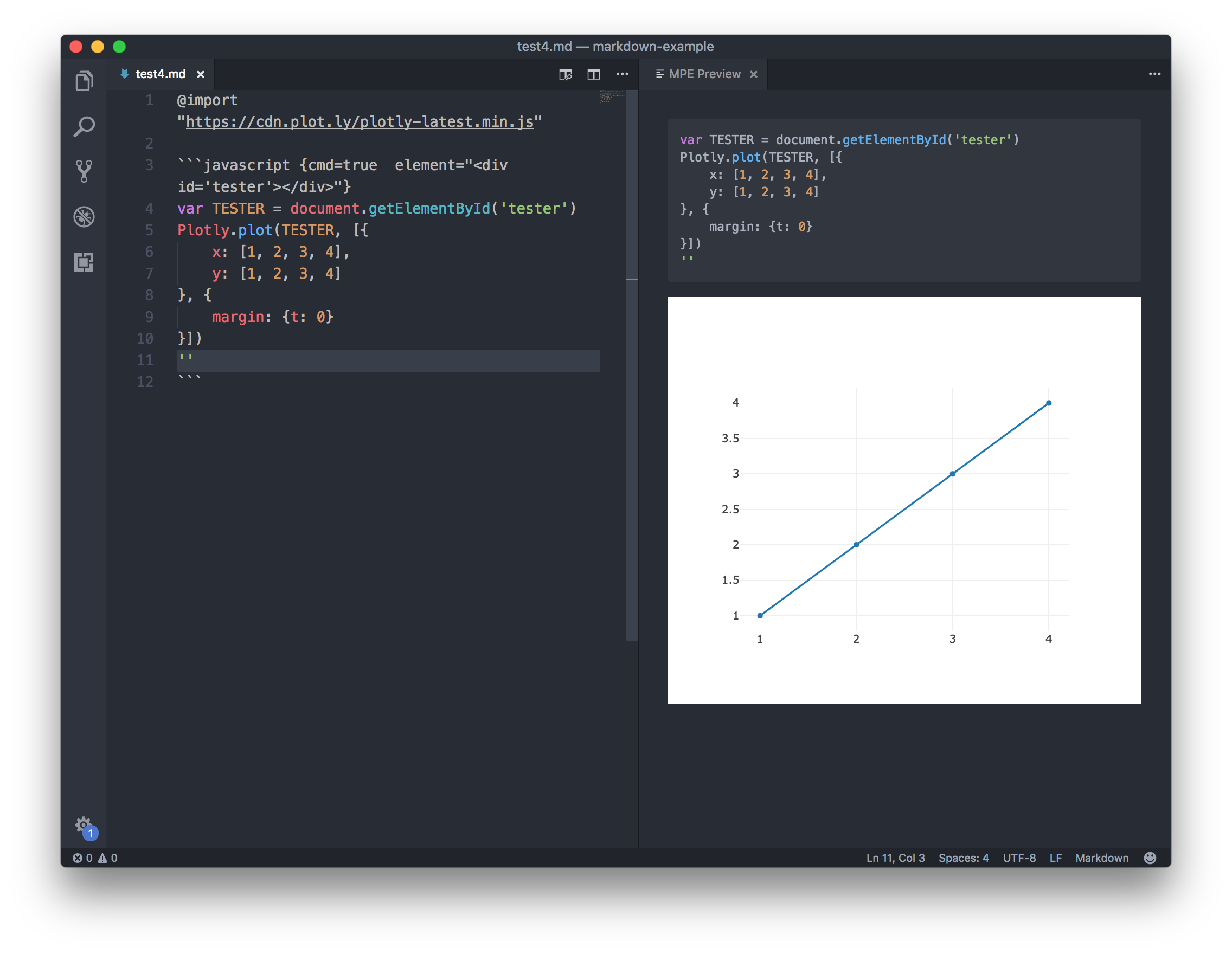• 第一行中的 @import "https://cdn.plot.ly/plotly-latest.min.js" 使用了 文件引用 的特性来引用 plotly-latest.min.js 文件。但是，引用本地的 js 文件是推荐的，因为这样更快。 • 接着我们创建了 javascript code chunk. Demo 下面的例子展示了如何利用 erd 库绘制 ER diagram。 1. erd {cmd=true output="html" args=["-i", "$input_file", "-f", "svg"]}
2. [Person]
3. *name
4. height
5. weight
6. +birth_location_id
7. [Location]
8. *id
9. city
10. state
11. country
12. Person *--1 Location
13. 

erd {cmd=true output="html" args=["-i", "\$input_file", "-f", "svg"]}

• erd 是我们将要用到的程序。 (当然你得先安装好这个程序)
• output="html" 意味着代码的输出结果将会被视作为 html
• args 显示了我们将要用到的参数。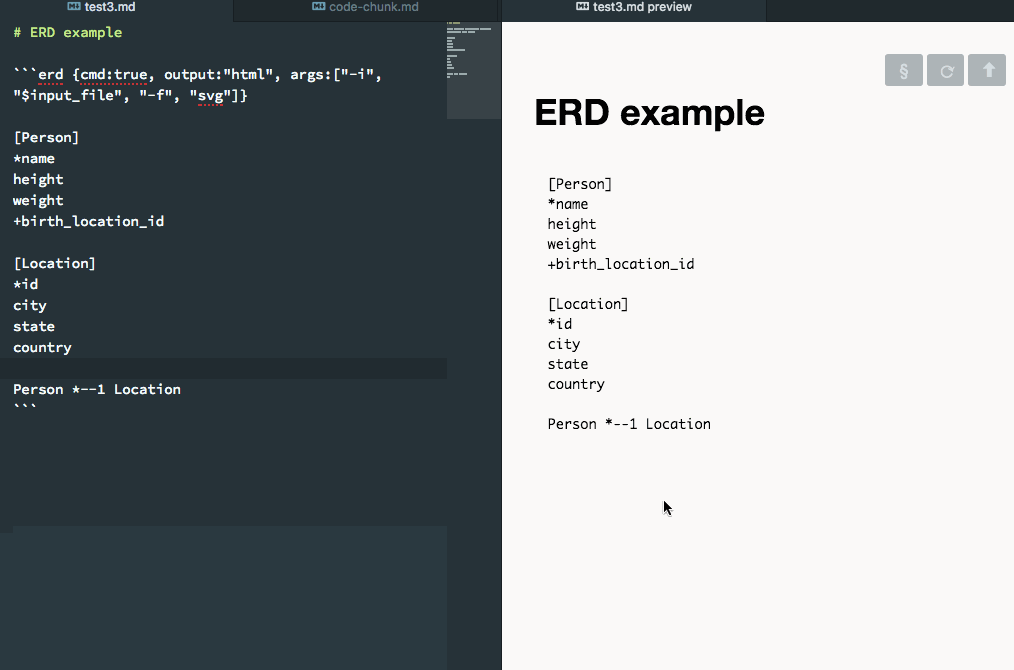展示

bashgnuplot with svg output局限

• 暂时不能在 ebook 工作。
• pandoc document export 中可能会有问题。

➔ 幻灯片制作